# Crimson Lynx

Captain Emily has a ship, the H. M. S Crimson Lynx. The ship is five furlongs from the dread pirate Umaima and her merciless band of thieves.
If her ship hasn't already been hit, Captain Emily has probability 3/5 of hitting the pirate ship. If her ship has been hit, Captain Emily will always miss.
If her ship hasn't already been hit, dread pirate Umaima has probability 1/7 of hitting the Captain's ship. If her ship has been hit, dread pirate Umaima will always miss.
If the Captain and the pirate each shoot once, and the pirate shoots first, what is the probability that the pirate misses the Captain's ship, but the Captain hits?

p =  0.5143

### Step-by-step explanation: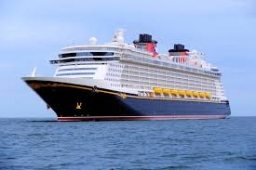Did you find an error or inaccuracy? Feel free to write us. Thank you!Tips to related online calculators
Need help to calculate sum, simplify or multiply fractions? Try our fraction calculator.
Would you like to compute count of combinations?

## Related math problems and questions:

• Three shooters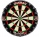Three shooters shoot, each one time, on the same target. The first hit the target with a probability of 0.7; second with a probability of 0.8 and a third with a probability of 0.9. What is the probability to hit the target: a) just once b) at least once c
• Shooter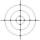The probability that a good shooter hits the center of the target circle I is 0.1. The probability that the target hit intercircle is II 0.58. What is the probability that it hits the target circle I or II?
• The shooter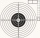The shooter shoots at the target, assuming that the individual shots are independent of each other and the probability of hitting each of them is 0.2. The shooter fires until he hits the target for the first time, then stop firing. (a) What is the most li
• Probability - tickets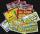What is the probability when you have 25 tickets in 5000 that you not wins the first (one) prize?
• Double probabilityThe probability of success of the planned action is 60%. What is the probability that we will achieve success at least once if this action is repeated twice?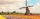How large must the group of people be so that the probability that two people have a birthday on the same day of the year is greater than 90%?
• Drawing from a hatWhen drawing numbers from a hat from 1 to 35, we select random given numbers. What is the probability that the drawn numbers will be divisible by 8 and 2?
• Duval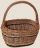Duval went to bat 80 times during a baseball season. In the first half of the season he had 15 hits. In the second half of the season he had 9 hits. Which percent of the time did Duval get a hit during the entire season?
• DicesWe will throw two dice. What is the probability that the ratio between numbers on first and second dice will be 1:2?
• We rollWe roll two dice A. - what is the probability that the sum of the falling numbers is at most 4 B. - is at least 10 C. - is divisible by 5?
• Dice and coinA number cube is rolled and a coin is tossed. The number cube and the coin are fair. What is the probability that the number rolled is greater than 2 and the coin toss is head?
• Balls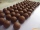From the bag with numbered balls (numbers 1,2,3,. ..20) we pick one ball. What is the probability of choosing a number containing 1?
• The elevation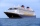The elevation of a sunken ship is -120 feet. Your elevation is 5/8 of the ship’s elevation. What is your elevation?
• Right key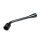The hostel has 4 rooms. The keys to each room are not numbered. Each of the four guests took one key. What is the probability that everyone took the right key?
• RecordsRecords indicate 90% error-free. If 8 records are randomly selected, what is the probability that at least 2 records have no errors?
• Ten dices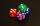When you hit ten dices at the same time you get average 35. How much do you hit if every time you get six, you're throwing the dice again?
• Probability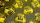What are the chances that in the lottery, in which the numbers are drawn 5 of 50, you win the first prize?